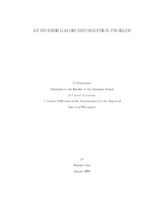## An Inverse Galois Deformation Problem##### Abstract
Suppose $\bar{\rho}: \Gal({\bar{F}/F}) \rightarrow \GL_2(\mathbf{k})$ is a residual Galois representation satisfying several mild conditions, where $F$ is a number field and $\mathbf{k}$ is a finite field with characteristics $p \geq 7$. In this work, we show that for any finite flat reduced complete intersection over $W(\mathbf{k})$, $\mathcal{R}$, we can construct a deformation problem defined by local conditions imposed on some finite set of places in $F$, such that the corresponding universal deformation ring of $\bar{\rho}$ is $\mathcal{R}$. It's a theorem of Wiles that if the local conditions are chosen to express restriction to deformations coming from modular forms, then the corresponding universal deformation ring is a finite flat reduced complete intersection, so our work can be regarded as a converse to Wiles' result.
2018-08-30
##### Keywords
Galois representation; number theory; universal deformation ring; Mathematics; deformation theory
##### Committee Chair
Ramakrishna, Ravi Kumar
##### Committee Member
Zywina, David J.
Templier, Nicolas P.
Mathematics
##### Degree Name
Ph. D., Mathematics
##### Degree Level
Doctor of Philosophy
##### Types
dissertation or thesis